Next: Rating floors Up: Details of the Rating Previous: Special rating formula

## Standard rating formula

This algorithm is to be used for players with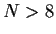who have not had either all wins or all losses in every previous rated game.

Define the Standard winning expectancy,''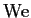, between a player rated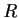and his/her-th opponent rated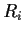to be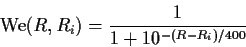The value of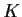, which used to take on the values 32, 24 or 16, depending only on a player's pre-event rating, is now defined as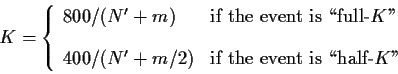where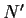is the effective number of games, and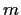is the number of games the player completed in the event. The following are example values offor particular values ofand.

 Value ofFull-K Half-K 6 4 80 50 6 6 66.67 44.44 6 10 50 36.36 20 4 33.33 18.18 20 6 30.77 17.39 20 10 26.67 16 50 4 14.81 7.69 50 6 14.29 7.54 50 10 13.33 7.27

If, or if the player competes against any opponent more than twice, the standard'' rating formula that results in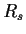is given by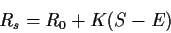where the player scores a total ofpoints (1 for each win, 0 for each loss, and 0.5 for each draw), and where the total winning expectancy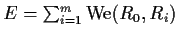.

If both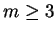and the player competes against no player more than twice, then the standard'' rating formula that results inis given by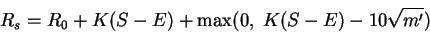where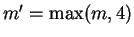(3-round events are treated as 4-round events when computing this extra term). The quantity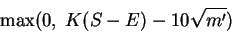is, in effect, a bonus amount for a player who performs unusually better than expected. Note that the value 10'' in the above formula will change to 16'' in January 2003.

The resulting value ofis the rating produced by the standard'' rating algorithm.Next: Rating floors Up: Details of the Rating Previous: Special rating formula
Mark Glickman
2004-09-22